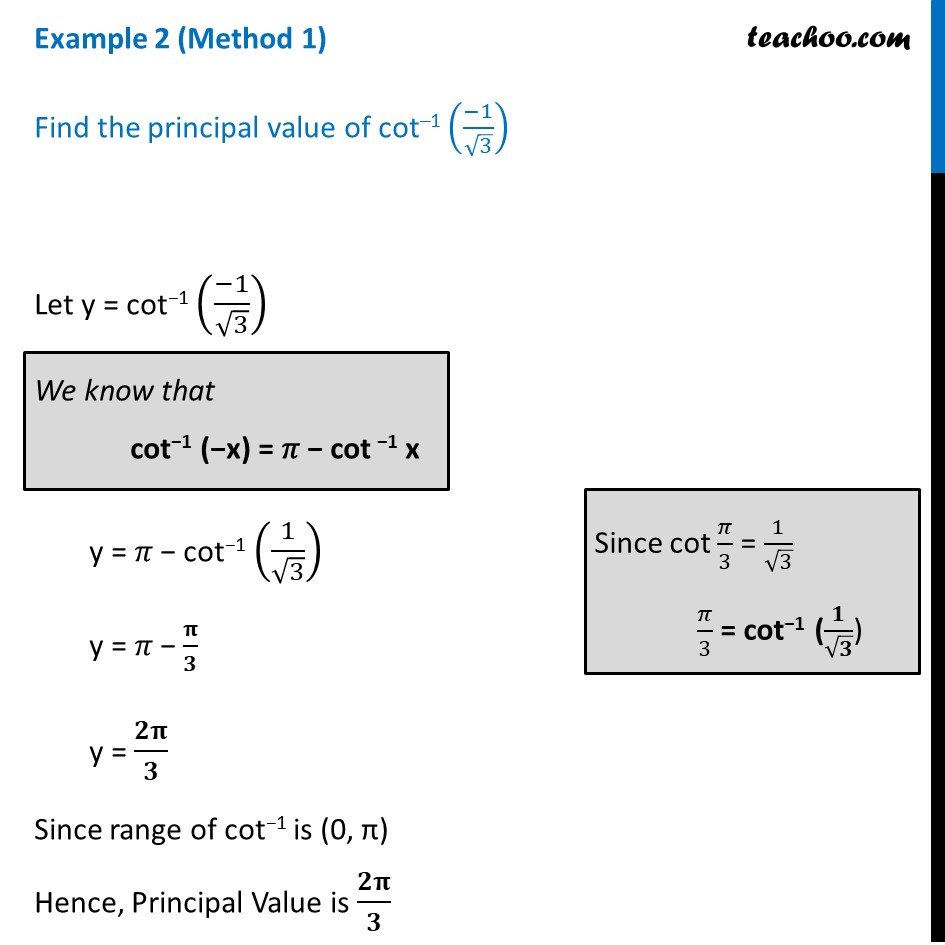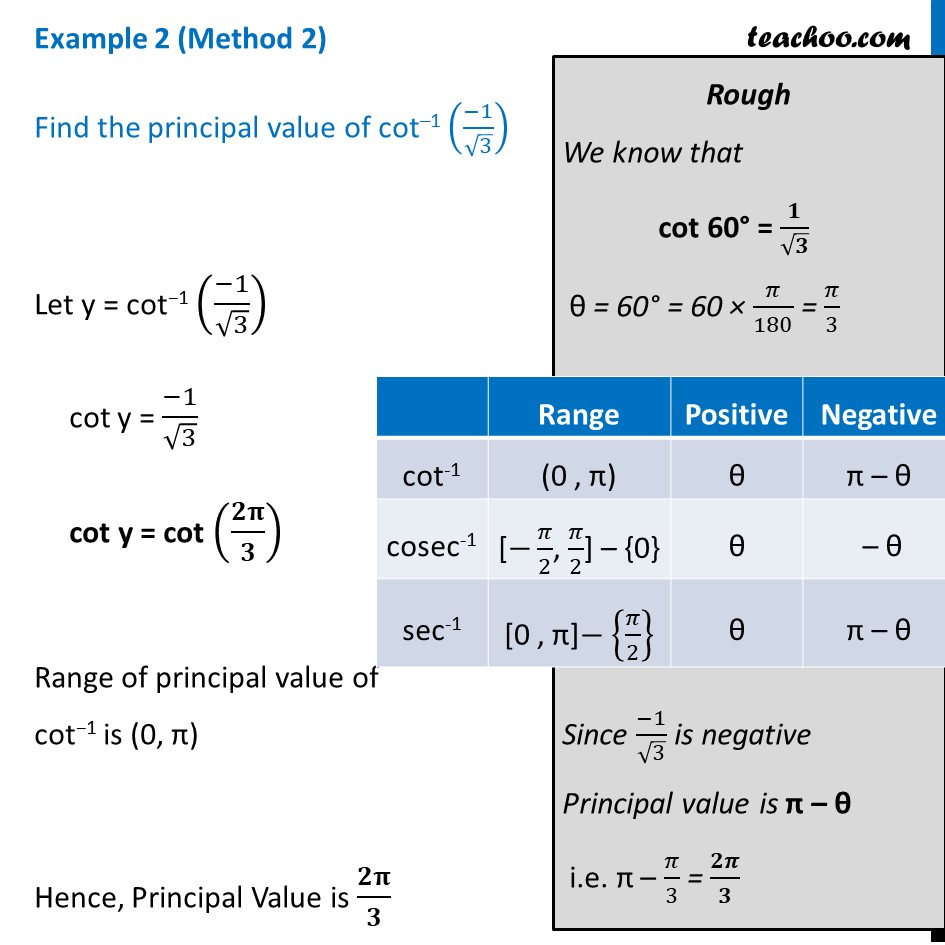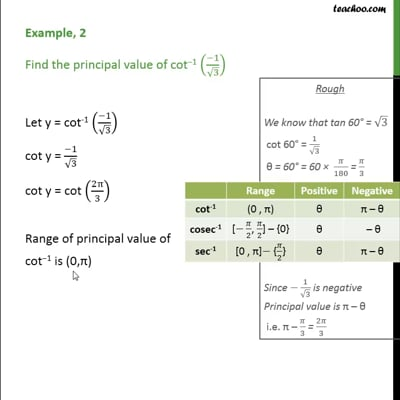Examples

Chapter 2 Class 12 Inverse Trigonometric Functions
Serial order wiseThis video is only available for Teachoo black users

Introducing your new favourite teacher - Teachoo Black, at only ₹83 per month

### Transcript

Example 2 (Method 1) Find the principal value of cot–1 ((−1)/√3) Let y = cot−1 ((−1)/√3) y = 𝜋 − cot−1 (1/√3) y = 𝜋 − 𝛑/𝟑 y = 𝟐𝛑/𝟑 Since range of cot−1 is (0, π) Hence, Principal Value is 𝟐𝛑/𝟑 We know that cot−1 (−x) = 𝜋 − cot −1 x Since cot 𝜋/3 = 1/√3 𝜋/3 = cot−1 (𝟏/√𝟑) Example 2 (Method 2) Find the principal value of cot–1 ((−1)/√3) Let y = cot−1 ((−1)/√3) cot y = (−1)/√3 cot y = cot (𝟐𝛑/𝟑) Range of principal value of cot−1 is (0, π) Hence, Principal Value is 𝟐𝛑/𝟑 Rough We know that cot 60° = 𝟏/√𝟑 θ = 60° = 60 × 𝜋/180 = 𝜋/3 Since (−1)/√3 is negative Principal value is π – θ i.e. π – 𝜋/3 = 𝟐𝝅/𝟑